# GSEB Solutions Class 8 Maths Chapter 7 Cube and Cube Roots InText Questions

Gujarat Board GSEB Textbook Solutions Class 8 Maths Chapter 7 Cube and Cube Roots InText Questions and Answers.

## Gujarat Board Textbook Solutions Class 8 Maths Chapter 7 Cube and Cube Roots InText Questions

Try These (Page 111)

Question 1.
Find the ones digit of the cube of each of the following numbers?

1. 3331
2. 8888
3. 149
4. 1005
5. 1024
6. 77
7. 5022
8. 53

Solution: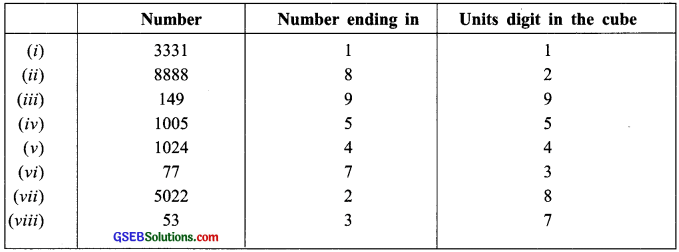Note:
1. There are only 4 numbers, less than 100 which are perfect cubes. They are:
1, 8, 27 and 64.

2. There are only 5 numbers between 100 and 1000 which are perfect cubes.
These are: 125, 216, 343, 512 and 729.

3. The least 4 digit perfect cube is 1000.Try These (Page 111)

Question 1.
Consider the following pattern:
1 = 13
3 + 5 = 8 = 23
7 + 9 + 11 = 27 = 33
13 + 15 + 17 + 19 = 64 = 43
21 + 23 + 25 + 27 + 29 = 125 = 52
Express the following numbers as the sum of odd numbers using the above pattern?
(a) 63
(b) 83
(c) 73
Solution:
(a) n = 6 and (n – 1) = 5
We start with (6 × 5) + 1 = 31 We have
63 = 31 + 33 + 35 + 37 + 39 + 41
= 216

(b) n = 8 and (n – 1) = 7
We have
83 = 57 + 59 + 61 + 63 + 65 + 67 + 69 + 71
= 512

(c) n = 7 and (n – 1) = 6
We have
73 = 43 + 45 + 47 + 49 + 51 + 53 + 55
= 343Question 2.
Consider the following pattern:
23 – 13 = 1 + 2 × 1 × 3
33 – 23 = 1 + 3 × 2 × 3
43 – 33= 1 + 4 × 3 × 3
Using the above pattern, find the value of the following:

1. 73 – 63
2. 123 – 113
3. 203 – 193
4. 513 – 503

Solution:
1. 73 – 63 = 1 + 7 × 6 × 3
= 1 + 126 = 127

2. 123 – 113 = 1 + 12 × 11 × 3
= 1 + 396 = 397

3. 203 – 193 = 1 + 20 × 19 × 3
= 1 + 1140 = 1141

4. 513 – 503 = 1 + 51 × 50 × 3
= 1 + 7650 = 7651

Note:
In the prime factorisation of any number, if each factor appears three times, then the number is a perfect cube.

Try These (Page 112)

Question 1.
Which of the following are perfect cubes?
1. 400
2. 3375
3. 8000
4. 15625
5. 9000
6. 6859
7. 2025
8. 10648
Solution:
1. We have
400 = 2 × 2 × 2 × 2 × 5 × 5
∵ 2 × 5 × 5 remain after grouping in triples.
400 is not a perfect cube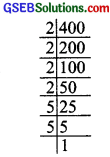2. We have 3375 = 3 × 3 × 3 × 5 × 5 × 53. We have 8000= 2 × 2 × 2 × 2 × 2 × 2 × 5 × 5 × 5
∵ The prime factors of 15625 can be grouped into triples and no factor is left over.
∴ 8000 is a perfect cube

4. We have
15625 = 5 × 5 × 5 × 5 × 5 × 5
∵ The prime factors of 15625 can be grouped into triples no factor is left over.
∴ 15625 is a pefect cube.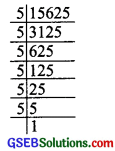5. We have
9000 = 2 × 2 × 2 × 3 × 3 × 5 × 5 × 5
The prime factors of 9000 cannot be grouped into triples (because 3 × 3) are left over.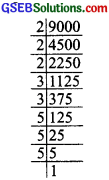∴ 9000 is not a perfect cube.

6. We have 6859 = 19 × 19 × 19
∵ The prime factors of 6859 can be grouped into triples and no factor is left over.
∴ 6859 is a perfect cube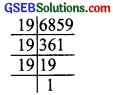7. We have
2025 = 3 × 3 × 3 × 3 × 5 × 5
∵ We do not get triples of prime factors of 2025 and 3 × 5 × 5 are left over.
∴ 2025 is not a perfect cube.8. We have
10648 = 2 × 2 × 2 × 11 × 11 × 11
∵ The prime factors of 10648 can be grouped into triples and no factor is left over.
∴ 10648 is a perfect cube.Try These (page 113)

Question 1.
Check which of the following are perfect cubes?
(i) 2700
(ii) 16000
(iii) 64000
(iv) 900
(v) 125000
(vi) 36000
(vii) 21600
(viii) 10000
(ix) 27000000
(x) 1000
What pattern do you observe in these perfect cubes?
Solution:
(i) We have
2700 = 2 × 2 × 3 × 3 × 3 × 5 × 5
We do not get complete triples of prime factors, i.e., 2 × 2 and 5 × 5 are left over.
∴ 2700 is not a perfect cube.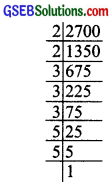(ii) We have
1600 = 2 × 2 × 2 × 2 × 2 × 2 × 5 × 5
After grouping in 3’s we get 5 × 5 which is ungrouped in triples.
1600 is not a perfect cube.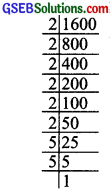(iii) We have
64000 = 2 × 2 × 2 × 2 × 2 × 2 × 2 × 2 × 2 × 5 × 5 × 5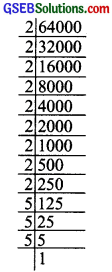Since, we get groups of triples.
∴ 64000 is a perfect cube.

(iv) We have
900 = 2 × 2 × 3 × 3 × 5 × 5
which are ungrouped in triples.
∴ 900 is not a perfect cube.(v) We have 125000 = 2 × 2 × 2 × 5 × 5 × 5 × 5 × 5 × 5
As we get all the prime factors in the group of triples.
∴ 125000 is a perfect cube.(vi) We have
36000 = 2 × 2 × 2 × 2 × 2 × 3 × 3 × 5 × 5 × 5
While grouping the prime factors of 36000 in triples, we are left over with 2 × 2 and 3 × 3.
∴ 36000 is not a perfect cube.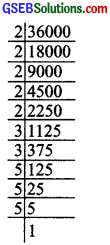(vii) We have
21600 = 2 × 2 × 2 × 2 × 2 × 3 × 3 × 3 × 5 × 5
While grouping the prime factors of 21600 in triples, we are left with 2 × 2 and 5 × 5.
∴ 21600 is not a perfect cube.(viii) We have
10000 = 2 × 2 × 2 × 2 × 5 × 5 × 5 × 5
While grouping the prime factors into triples, we are left over with 2 and 5.
∴ 10000 is not a perfect cube.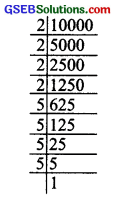(ix) We have 27000000 = 2 × 2 × 2 × 2 × 2 × 2 × 3 × 3 × 3 × 5 × 5 × 5 × 5 × 5 × 5
Since, all the prime factors of 27000000 appear in groups of triples.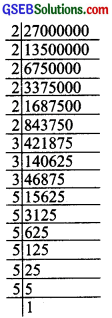27000000 is a perfect cube.

(x) We have
1000 = 2 × 2 × 2 × 5 × 5 × 5
Since, all the prime factors of 1000 appear in groups of triples.
∴ 1000 is a perfect cube.
Now, in these perfect cubes, we can say that “the number of zeros at the end of a perfect cube must be 3 or a multiple of 3, failing which the number cannot be a perfect cube.”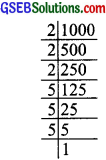Try These (Page 115)

Question 1.
State true or false: for any integer m, m2 < m3. Why?
Solution:
It is not always true.
For example, let m = -1
We have m2 = (-1)2 = 1
and m3 = (-1)3 = -1
∴ The above statement, i.e., m2 < m3 is false.